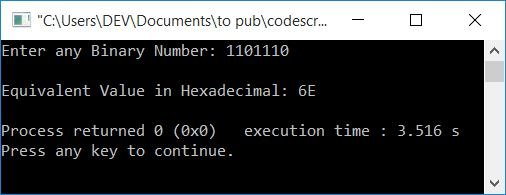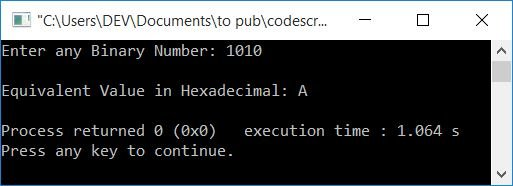# C++ Program to Convert Binary to Hexadecimal

In this article, you will learn and get code for binary to hexadecimal conversion in C++. The program is created with and without using user-defined functions.

But before going through the program, if you are not aware of the steps and formula used for binary to hexadecimal conversion, then you can go there to get all the things you need.

## Binary to Hexadecimal in C++

To convert a binary number to hexadecimal in C++ programming, you have to ask the user to enter any number in the binary number system. Then convert it into its equivalent hexadecimal value. Print the hexadecimal value as output.

The question is: write a program in C++ to receive any binary number and print its equivalent hexadecimal value.  The answer to this question is given below:

```#include<iostream>
using namespace std;
int main()
{
int binaryNum, hex=0, mul=1, chk=1, rem, i=0;
char hexDecNum;
cout<<"Enter any Binary Number: ";
cin>>binaryNum;
while(binaryNum!=0)
{
rem = binaryNum%10;
hex = hex + (rem*mul);
if(chk%4==0)
{
if(hex<10)
hexDecNum[i] = hex+48;
else
hexDecNum[i] = hex+55;
mul = 1;
hex = 0;
chk = 1;
i++;
}
else
{
mul = mul*2;
chk++;
}
binaryNum = binaryNum/10;
}
if(chk!=1)
hexDecNum[i] = hex+48;
if(chk==1)
i--;
for(i=i; i>=0; i--)
cout<<hexDecNum[i];
cout<<endl;
return 0;
}```

This program was built and runs under the Code::Blocks IDE. Here is its sample run:Now supply the binary number input, say 1101110, and press the ENTER key to convert it to its equivalent hexadecimal value as shown in the snapshot given below:Here is another sample run with user input as 1010:The dry run of the above program with user input 1010 goes like this:

• Initial values, hex = 0, mul = 1, chk = 1, i = 0
• When the user enters 1010 as a binary input, then 1010 gets initialized to binaryNum. So binaryNum=1010
• Now the condition of the while loop, binaryNum!=0 or 1010!=0, evaluates to be true. Therefore, program flow goes inside the loop.
• binaryNum%10 or 1010%10 or 0 is set to rem. So rem=0
• hex+(rem*mul) or 0+(0*1) or 0 gets initialized to hex. So hex=0
• Now the condition of the if statement, that is, chk%4==0 or 1%4==0, evaluates to be false. As a result, program flow does not goes inside the if's body, but rather to else's body.
• And mul*2 or 1*2 or 2 gets initialized to mul. Now mul=2
• And then the value of chk gets incremented. Now chk=2
• And at last (the last statement of of while's body), binaryNum/10 or 1010/10 or 101 gets initialized to binaryNum. Now binaryNum=101
• Now the program flow goes back and evaluates the condition of the while loop again with the new value of binaryNum. Again, the condition 101!=0 is evaluated to be true. Therefore, again, program flow goes inside the loop.
• Process the code until the while loop's condition evaluates to false.
• On continuing the process, here are the values we'll get after each evaluation of the while loop:
• After the first evaluation
rem=0, hex=0, mul=2, chk=2, binaryNum=101
• After a second evaluation
rem=1, hex=2, mul=4, chk=3, binaryNum=10
• After the third evaluation
rem=0, hex=2, mul=8, chk=4, binaryNum=1
• After the fourth evaluation, this time the condition of the if statement, that is, chk%4==0 or 4%4==0 or 0==0, evaluates to be true.
rem=1, hex=0, hexDecNum=A, mul=1, chk=1, i=1, binaryNum=0
• Now, after the fourth evaluation, the value of binaryNum is 0. As a result, the condition binaryNum!=0 or 0!=0 evaluates to false. Therefore, program flow does not goes inside the loop this time. It means that the while loop's evaluation is completed.
• If the condition chk!=1 or 1!=1 is evaluated after the while loop, it is now false.
• Now the program flow goes to the next if's condition, and evaluates its condition, that is, chk ==1 or 1 ==1 , which is evaluated to be true. So the value of i gets decremented. Now i=0
• Using a for loop, that starts from the value of i and continues until its value becomes equal to 0, print the value hexDecNum[] one by one.
• Because the value of i is 0, the loop only evaluates at once. As a result, when the value of hexDecNum is printed, it equals A.

### Binary to hexadecimal conversion in C++ using a user-defined function

Here is another program that also converts any binary number entered by the user at run-time into its equivalent hexadecimal value, but using a user-defined function called BinaryToHexaDec().

```#include<iostream>
using namespace std;

int i=0;
char hexDecNum;
int main()
{
int binaryNum;
cout<<"Enter any Binary Number: ";
cin>>binaryNum;
for(i=i; i>=0; i--)
cout<<hexDecNum[i];
cout<<endl;
return 0;
}
{
int hex=0, mul=1, chk=1, rem;
while(bin!=0)
{
rem = bin%10;
hex = hex + (rem*mul);
if(chk%4==0)
{
if(hex<10)
hexDecNum[i] = hex+48;
else
hexDecNum[i] = hex+55;
mul = 1;
hex = 0;
chk = 1;
i++;
}
else
{
mul = mul*2;
chk++;
}
bin = bin/10;
}
if(chk!=1)
hexDecNum[i] = hex+48;
if(chk==1)
i--;
}```

This program will produce the same output as the previous program.

#### The same program in different languages

C++ Quiz

« Previous Program Next Program »